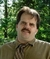## How would you calculate, using the spreadsheets statistical functions, the cheapest option at the fastest speed with the least downtime?Tags:
Microsoft Excel
microsoft excel functions
Microsoft Excel macros
i have 5 tables each including speed, price, download limit, average downtime and the average uptime. i was asked by my teacher to calculate all these so i get one number, to find out which is the best value for money for someone to go for. i just need to know what do i calcute to what to get my answers and which letters or numbers i type into excel to get the answer becasue i need to work it out from there.

Thanks. We'll let you know when a new response is added.
•